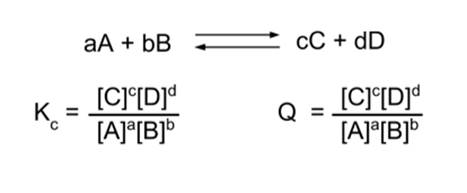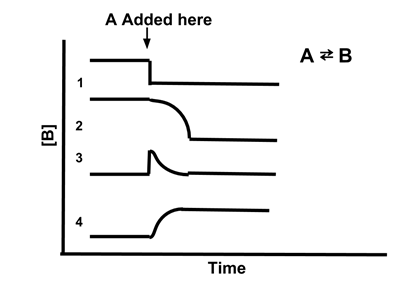# Enduring Understanding 6.B: Le Chatelier's Principle

• Chemical equilibrium is a dynamic equilibrium - the forward and reverse reactions are occurring, but at an equal rate so the concentrations of reactants and products do not change.
• Changing conditions - adding or removing reactants or products, changing temperature, pressure, or concentration - can result in the system no longer being in equilibrium.
• This is often referred to as a 'stress' on the system.
• The reaction will then occur until equilibrium is restored.

• Le Chatelier's Principle states that, when a system at equilibrium is stressed by a change in conditions, the system will adjust itself to counteract the change and re-establish equilibrium.
• Example: Suppose the following reaction is at equilibrium.
•• Equilibrium means that Kc = Q.
• Now, stress the system by doubling the concentration of A, [A].
• Q has dropped ([A] is in the denominator) and now is no longer equal to Kc.
• Now, Kc > Q. This means the reaction will proceed in the forward direction.
• Therefore, the reaction proceeds in the forward direction until equilibrium is established.
• Similarly, if we instead double the concentration of [C], Kc < Q and the reaction will proceed in the reverse direction until equilibrium is established.
• Therefore, Le Chatelier's Principle predicts that if we add a reagent to a reaction system at equilibrium, the reaction will proceed in the forward direction; and if we add a product, it will proceed in the reverse direction.
• Changing pressure can also affect chemical equilibrium, in reactions where there is a different number of reactant and product particles in the gas phase. Higher pressure favors fewer particles.
• Example:
• N2(g) + 3H2(g) → 2NH3(g)
• This reaction has four particles on the reactant side, two on the product side. Increasing the pressure favors the side with fewer particles - so increasing the pressure shifts the equilibrium in favor of the products.
• Changing temperature can affect equilibrium constants, depending on whether the reaction is endothermic or exothermic.
• Endothermic reactions are favored by an increase in temperature, so heating an endothermic reaction will shift equilibrium towards the products.
• Exothermic reactions are favored by a decrease in temperature, so cooling an exothermic reaction will shift equilibrium towards the products.
• Sometimes, catalysts are added to a reaction to increase the rate of the reaction. Remember that catalysts increase the rate of reactions, but have no effect on the equilibrium constant itself. So adding a catalyst will never change an equilibrium, it will just allow equilibrium to be reached more quickly.
• Sample Problem: Which line best illustrates the concentration of [B] if A is added to the reaction A ⇆ B?
•• Answer: (4). Adding A increases the amount of the reactant, so the reaction will shift to restore equilibrium. This will increase the concentration of A, shifting Qc to be less than Kc. Therefore, [B] will increase to restore the equilibrium. Only line 4 shows [B] increasing when A is added.

 Related Links: Chemistry Chemistry Quizzes AP Chemistry Notes Equilibrium Problems

To link to this Le Chatelier's Principle page, copy the following code to your site: